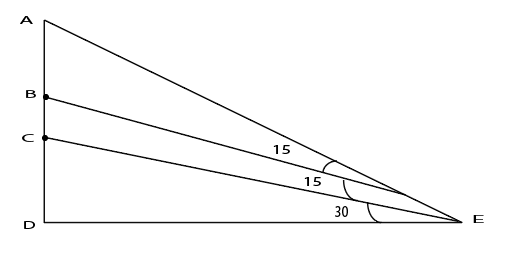Question 25

# The topmost point of a perfectly vertical pole is marked A. The pole stands on a flat ground at point D. The points B and C are somewhere between A and D on the pole. From a point E, located on the ground at a certain distance from D, the points A, B and C are at angles of 60, 45 and 30 degrees respectively. What is AB : BC : CD?

SolutionLet ED = $$\sqrt{\ 3}x$$
In triangle CDE, $$\tan\ 30\ =\ \frac{CD}{\sqrt{\ 3}x}$$ => CD = x

In triangle BDE, $$\tan\ 45\ =\ \frac{BD}{\sqrt{\ 3}x}$$ => BD = $$\sqrt{\ 3}x$$ => BC = $$\sqrt{\ 3}x\ -x$$

In triangle ADE, $$\tan\ 60\ =\ \frac{AD}{\sqrt{\ 3}x}$$ => AD = 3x => AB = $$3x\ -\ \sqrt{\ 3}x$$

AB : BC : CD = $$(3 - \sqrt 3) : (\sqrt 3 - 1) : 1$$